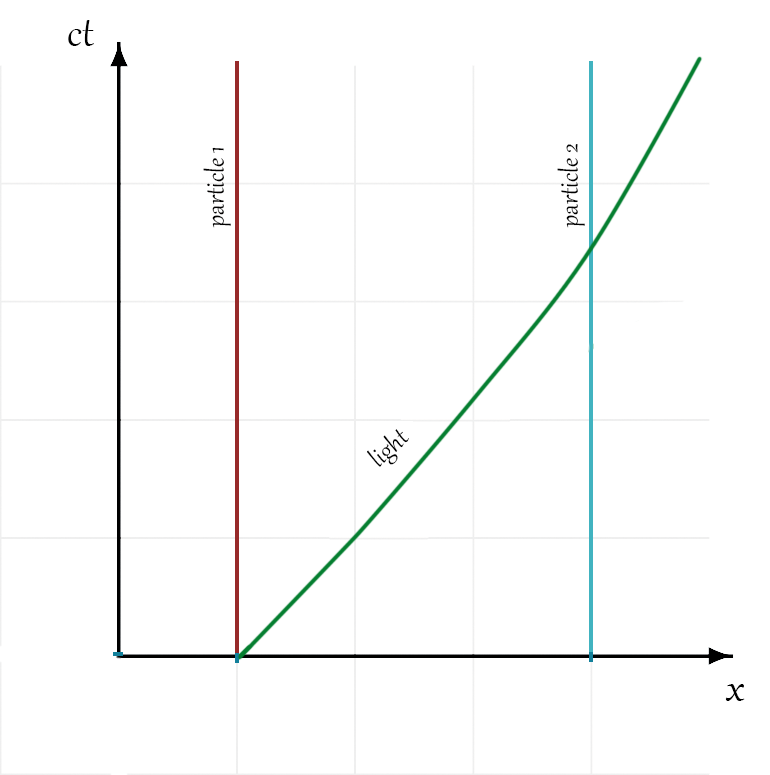$$\require{cancel}$$

# Exercise 5.1.1We cheated a bit here and made a $$x$$ vs. $$ct$$ plot so that a particle moving at the speed of light has a slope of $$1$$.

# Exercise 5.2.1

"at time $$t=t_1$$" so $$dt = 0$$, so $$ds = a(t)dx$$. Since the ruler is at rest in the given coordinate system its length is indeed given by $$ds$$ at time $$t_1$$. Therefore the length of the ruler is $$ds = a(t_1)dx_1$$.

# Exercise 5.3.1

"constant $$x$$" so $$dx = 0$$, and then $$ds = cdt$$. Therefore the time elapsed on the clock is

\begin{equation*} \begin{aligned} \int \frac{1}{c}\sqrt{-ds^2} =\int_{t_1}^{t_2} dt = t_2 - t_1. \end{aligned} \end{equation*}

# Exercise 5.4.1

See solution to Exercise 5.4.2

# Exercise 5.4.2Since the amount of distance corresponding to a given $$dx$$ is changing with time, the slope of the photon's world line is changing with time.

# Exercise 5.5.1

Substituting in $$dt = a(t)d\tau$$ to Equation 5.2 and factoring out $$a^2(t)$$ gives us

\begin{equation*} \begin{aligned} ds^2 = -c^2a^2(t)[d\tau^2 + dx^2]. \end{aligned} \end{equation*}

Assuming a one-to-one correspondence between $$t$$ and $$\tau$$ (which one would have in an expanding universe given definition of $$d\tau$$) we can use $$a(\tau) \equiv a(t(\tau))$$ in its place and write

\begin{equation*} \begin{aligned} ds^2 = a^2(\tau)[-c^2d\tau^2 + dx^2] \end{aligned} \end{equation*}

# Exercise 5.6.1